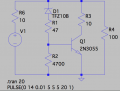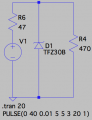need small help in the circuit

RenesasT1

Joined Jul 6, 2017
107
Hello ,

how the following condition can be done?

One of my IC requires power Vb. If my external power supply is set to 12V then Vb of IC should be 12V.

If my external power supply is set to 20V then Vb of IC should be 20V.

If my external power supply is set to 28V then Vb of IC should be 28V.

If my external power supply is set to 29V then Vb of IC should be 29V.

If my external power supply is set to 30V then Vb of IC should be 29V.

If my external power supply is set to 32V then Vb of IC should be 29V.

If my input is between 8V to 29V then same should be input to the IC i.e. Vb. if input is greater than 29v lets say 29.1 V to 32.5, the voltage input to IC should be 29.

wayneh

Joined Sep 9, 2010
17,160
With several guesses about what you need, I'll suggest a 29V zener diode at the Vb input of the IC. It will do nothing until the voltage there exceeds 29V. Above that, it will conduct current to ground. So you'll also need a resistor in series with Vb, so that the current conducted by the zener can drop the voltage seen on the IC's power pin.

This solution may not be practical if the IC needs a lot of current.

•#12

tribbles

Joined Jun 19, 2015
28
I used a LTC7812 for a PSU - it's a buck/boost PSU chip that you can do what you want - the output voltage can follow the input voltage; if it's too high, then it'll buck the voltage down; if it's too low then it boosts it.

In my case, the range was 12.5V to 18.9V (for a CPU that needed 12V to 19V), and 65W capability. The input voltage was from 10V to 32V (although it should work outside this range)

Not the world's smallest circuit though - and there is a mistake in their reference design (it feeds the output voltage back into itself for efficiency - but you can't do this if the output voltage is above ~14V [EXTVCC]. Leave this disconnected if your maximum voltage is over 14V [which yours is]).

crutschow

Joined Mar 14, 2008
28,474
Instead of a 29V zener for wayneh's shunt regulator you could use a TL431 adjustable reference (zener).
It's more stable and you can adjust it to exactly the voltage you want.

How much current does the IC need?

RenesasT1

Joined Jul 6, 2017
107
Hello all,

The IC requires 50mA of current.

The main point is the operating voltage. The operating voltage (Vb) is user dependable i.e from 8V to 32.5V. Suppose Vb is 8V then it should not affect on 8V . If it gets affected to 8V then IC will not work.

I need a solution which will impact voltage only over the 29.5V.

crutschow

Joined Mar 14, 2008
28,474
What's the maximum difference you can tolerate between the input voltage and the voltage to the IC if it's less than 20.5V?
An answer of zero volts is not achievable.

wayneh

Joined Sep 9, 2010
17,160
An answer of zero volts is not achievable.
Not easily, no, but anything is possible at a cost.

wayneh

Joined Sep 9, 2010
17,160
I need a solution which will impact voltage only over the 29.5V.
Here's one example - a circuit I had on hand. It shows how to limit the voltage at R4 to just 10V. It's not perfect (which relates to @crutschow 's question) but works. My first zener idea follows below. I didn't tweak the values - this is just a gross description.

These are for nothing if you cannot tolerate some small voltage impact below the set point voltage.Last edited:

RenesasT1

Joined Jul 6, 2017
107
If voltage is 28 , I need 28 i/p to IC.

If voltage is 30, I need 29.5 i/p to IC.

If voltage is 32, I need 29.5 i/p to IC

If voltage is 29.5, I need 29.5 i/p to IC

If voltage is 27, I need 27 i/p to IC.